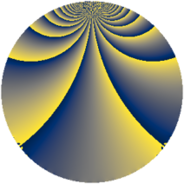# Properties

 Label 1470.2.vLevel $1470$ Weight $2$ Character orbit 1470.v Rep. character $\chi_{1470}(313,\cdot)$ Character field $\Q(\zeta_{12})$ Dimension $160$ Sturm bound $672$

# Related objects

## Defining parameters

 Level: $$N$$ $$=$$ $$1470 = 2 \cdot 3 \cdot 5 \cdot 7^{2}$$ Weight: $$k$$ $$=$$ $$2$$ Character orbit: $$[\chi]$$ $$=$$ 1470.v (of order $$12$$ and degree $$4$$) Character conductor: $$\operatorname{cond}(\chi)$$ $$=$$ $$35$$ Character field: $$\Q(\zeta_{12})$$ Sturm bound: $$672$$

## Dimensions

The following table gives the dimensions of various subspaces of $$M_{2}(1470, [\chi])$$.

Total New Old
Modular forms 1472 160 1312
Cusp forms 1216 160 1056
Eisenstein series 256 0 256

## Trace form

 $$160q - 24q^{5} + O(q^{10})$$ $$160q - 24q^{5} - 12q^{10} - 8q^{11} - 8q^{15} + 80q^{16} - 24q^{22} + 24q^{23} + 24q^{25} + 24q^{26} + 16q^{30} + 48q^{31} - 12q^{33} - 160q^{36} + 8q^{37} + 48q^{38} + 80q^{43} + 8q^{46} + 24q^{47} - 16q^{51} + 80q^{53} + 32q^{57} + 44q^{58} - 48q^{61} - 64q^{65} + 80q^{67} + 96q^{71} + 24q^{73} + 48q^{75} + 32q^{78} - 24q^{80} + 80q^{81} - 48q^{82} - 64q^{85} + 32q^{86} - 60q^{87} - 12q^{88} - 48q^{92} - 16q^{93} - 40q^{95} + O(q^{100})$$

## Decomposition of $$S_{2}^{\mathrm{new}}(1470, [\chi])$$ into newform subspaces

The newforms in this space have not yet been added to the LMFDB.

## Decomposition of $$S_{2}^{\mathrm{old}}(1470, [\chi])$$ into lower level spaces

$$S_{2}^{\mathrm{old}}(1470, [\chi]) \cong$$ $$S_{2}^{\mathrm{new}}(35, [\chi])$$$$^{\oplus 8}$$$$\oplus$$$$S_{2}^{\mathrm{new}}(70, [\chi])$$$$^{\oplus 4}$$$$\oplus$$$$S_{2}^{\mathrm{new}}(105, [\chi])$$$$^{\oplus 4}$$$$\oplus$$$$S_{2}^{\mathrm{new}}(210, [\chi])$$$$^{\oplus 2}$$$$\oplus$$$$S_{2}^{\mathrm{new}}(245, [\chi])$$$$^{\oplus 4}$$$$\oplus$$$$S_{2}^{\mathrm{new}}(490, [\chi])$$$$^{\oplus 2}$$$$\oplus$$$$S_{2}^{\mathrm{new}}(735, [\chi])$$$$^{\oplus 2}$$